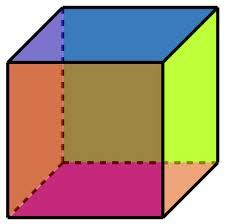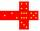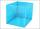# Cube

The sum of lengths of cube edges is 57 cm. What is its surface and volume?

S =  135.4 cm2
V =  107.2 cm3

### Step-by-step explanation:Did you find an error or inaccuracy? Feel free to write us. Thank you!Tips to related online calculators
Tip: Our volume units converter will help you with the conversion of volume units.

## Related math problems and questions:

• Magnified cubeIf the lengths of the cube's edges are extended by 5 cm, its volume will increase by 485 cm3. Determine the surface of both the original and the magnified cube.
• Cube edgesThe sum of the lengths of the cube edges is 42 cm. Calculate the surface of the cube.
• Sum of the edgesThe sum of the lengths of all edges of the cube is 72 cm. How many cm2 of colored paper are we going to use for sticking?
• Cube surfce2volumeCalculate the volume of the cube if its surface is 150 cm2.
• Cube 1-2-3Calculate the volume and surface area of the cube ABCDEFGH if: a) /AB/ = 4 cm b) perimeter of wall ABCD is 22 cm c) the sum of the lengths of all edges of the cube is 30 cm.
• The cubeThe cube has a surface of 600 cm2. What is its volume?
• Edges or sidesCalculate the cube volume, if the sum of the lengths of all sides is 276 cm.
• The cubeThe surface of the cube is 150 square centimeters. Calculate: a- the content of its walls b - the length of its edges
• Cuboid to cubeA cuboid with dimensions of 9 cm, 6 cm, and 4 cm has the same volume as a cube. Calculate the surface of this cube.
• Cube 6Volume of the cube is 216 cm3, calculate its surface area.
• The cubeThe cube has a surface area of 486 m ^ 2. Calculate its volume.
• Cube edgeDetermine the edges of the cube when the surface is equal to 37.5 cm square.
• Prism XThe prism with the edges of the lengths x cm, 2x cm, and 3x cm has volume 20250 cm3. What is the area of the surface of the prism?
• Cube 7Calculate the volume of a cube, whose sum of the lengths of all edges is 276 cm.
• Cube 5The surface of the cube is 15.36 dm2. How will change the surface area of this cube if the length of the edges is reduced by 2 cm?
• Cube surface and volumeThe surface of the cube is 500 cm2, how much cm3 will be its volume?
• CubeOne cube has an edge increased five times. How many times will larger it's surface area and volume?